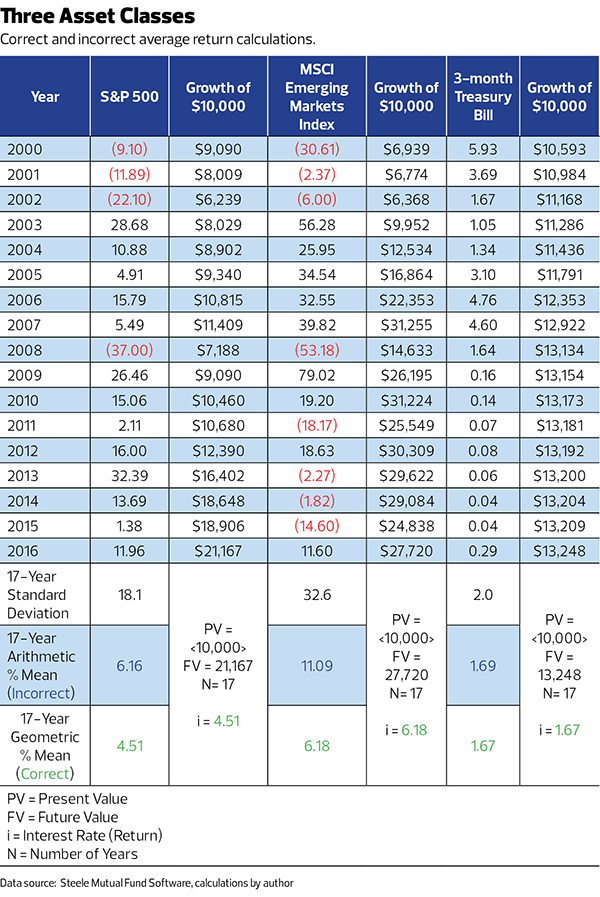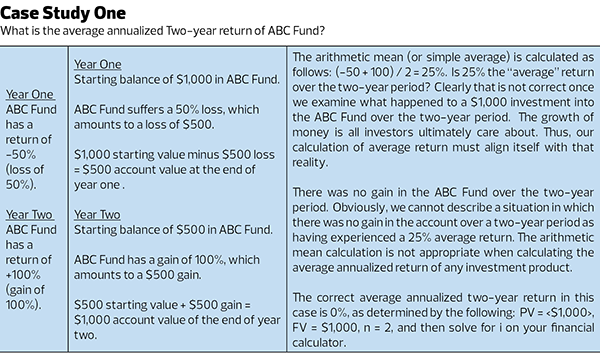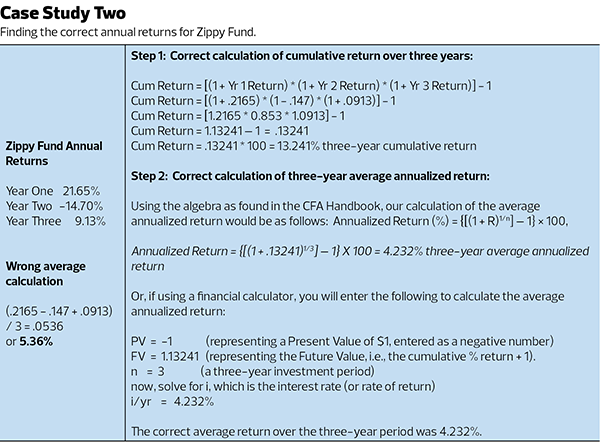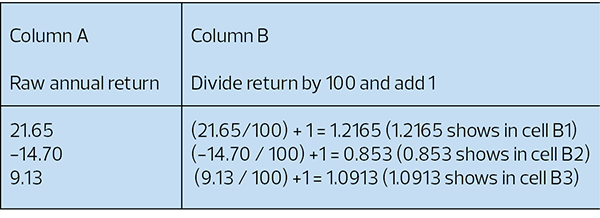# Get it right when it comes to calculating returns

Share
Register now

Many advisers seldom — if ever — take the time to determine the return of investments on their own. Often, they will rely on third-party calculations for the average annualized performance of funds and stocks.

That isn’t necessarily a problem, because high-quality data providers always use the geometric mean when showing “average annualized returns.” The problem may arise when you are called upon to calculate an average return of an investment over a multi-year period.

Here’s a bold statement for all advisers: If you are called upon to calculate the “average” return of an investment, make sure you use the geometric rather than the arithmetic mean.
Doing the math wrong means your clients will not fully understand their investment returns – and it’s adviser jail time for you. …

…okay, jail time may not happen. But, misrepresenting performance can certainly result in a fine.

Here’s why.The calculation for the arithmetic mean is simply the sum of all the numbers divided by total number count. It can never be an accurate guide for investment returns over the years, unless there is zero volatility, which is a next-to-impossible scenario.

The geometric mean, on the other hand, takes into account compounding — the returns compound upon each other. The arithmetic mean ignores compounding. In other words, the geometric mean recognizes the process by which investments actually grow or decline, which is geometric in nature, not arithmetic.

You must calculate the geometric mean to get a truly precise look at how a fund or stock has performed over time.

Here’s how it works.

THE WHY AND HOW
The following statements are found in the “Global Investment Performance Standards Handbook,” 3rd Edition, 2012, published by the CFA Institute:

Section 2: Calculation Methodology (page 15)
2.A.2. Firms must calculate time-weighted rates of return that adjust for external cash flows. Both periodic and sub-period returns must be geometrically linked.

Section 3-5 Provision 5.B.2.d (page 204)
… Annualized returns represent the geometric average annual compound return achieved over the defined period of more than one year. Annualized performance is only permitted for periods of one year or more.

Annualized Return (%) = {[(1 + R)1/n] – 1} × 100, where R is the cumulative return for the period, which is calculated by geometrically linking the sub-period returns during the period, and n is the number of years in the period.

Not recognizing the difference between an arithmetic mean and a geometric mean is where the problem begins.

INDEX PERFORMANCE
Let’s consider the returns of the S&P 500, the MSCI Emerging Markets Index and the three-month Treasury bill from 2000 through 2016 (see “Three Asset Classes”).Per the chart, the correct 17-year average annualized return (or geometric mean) for the S&P 500 is 4.51%, whereas the incorrect arithmetic average return is the significantly higher 6.16%.

The next column displays the annual growth of \$10,000. The terminal value by the end of 2016 is \$21,167. The correct growth rate (or average annualized percentage return) that turns the \$10,000 into \$21,167 is 4.51%, not 6.16%.

As clearly illustrated, the geometric mean is the rate of return that correctly expresses the growth of \$10,000. The arithmetic mean dramatically overstates the actual annualized growth rate of \$10,000. For this reason, the arithmetic mean is always incorrect when expressing the rate at which invested money grows over time.

Also worth noting is the fact that the gap between the incorrect arithmetic mean and the correct geometric mean widens as the standard deviation of return increases. For example, the difference between the two means is very small for the 3-month Treasury bill (1.69% arithmetic mean vs. 1.67% geometric mean). But the standard deviation of the 17 annual T-bill returns is also very small, at 2%.

By contrast, the fluctuation of returns for the MSCI EM Index is huge, as evidenced by the standard deviation of 32.6%. Accordingly, the difference between the arithmetic mean (11.09%) and geometric mean (6.18%) is quite large.

CASE STUDY ONE
Now we will examine two case studies to illustrate how to calculate the correct average return of an investment. Our first simple example will illustrate the problem of representing the arithmetic mean as the average return.CASE STUDY TWO
The case of the missing three-year average annualized return for the Zippy Fund. The Zippy Fund had a cumulative return of 13.241% over the three-year period.

With that calculated, we now can compute the three-year average annualized return (or the geometric mean). To invoke a driving metaphor, the cumulative percentage return represents the total distance traveled over three years, whereas the average annualized percentage return is the rate of speed traveled per year.The correct geometric mean will always be smaller than the incorrect arithmetic mean—precisely because the geometric mean is taking compounding into account, whereas the arithmetic mean fails to do so.

To make matters worse, the arithmetic mean becomes more wrong as the annual returns of the asset being analyzed are more volatile, as we observed in “Three Asset Classes.”

USING EXCEL TO CALCULATE THE GEOMETRIC MEAN
If you choose to perform this type of calculation in Excel, you can calculate the average annualized percentage return directly without first calculating the three-year cumulative percentage return.

The first step is to convert the raw returns into a format that Excel will accept when using the Geomean formula. You will need to divide each raw return by 100 and then add 1, as follows: (using the returns of the Zippy Fund):This transformation of the raw annual return data allows the Geomean function to work correctly, inasmuch as the Geomean function in Excel cannot work with negative numbers. To solve this, we express the negative return of -14.70% in year two as 0.853.

Thus, in this transformed format, positive annual returns will be represented by a number over 1 (such as 1.2165). A return of 0% will be represented as 1 exactly, and negative annual returns will be transformed into a number less than 1.

Now you are ready to use the “Geomean” function in Excel. In cell B4, you would enter the following formula: = Geomean (B1:B3)-1. The result will display as 0.0423. If you change the cell format to %, the result will display as 4.23%.

The bottom line, no matter which method you use, is this: When you’re tempted to calculate an average return, always, always, always think geometrically. Use any other method and you’ll be wrong.

Share